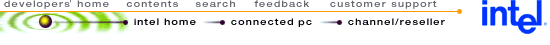## Using MMX™ Instructions to Perform Complex 16-Bit FFT

 Disclaimer Information in this document is provided in connection with Intel products. No license, express or implied, by estoppel or otherwise, to any intellectual property rights is granted by this document. Except as provided in Intel's Terms and Conditions of Sale for such products, Intel assumes no liability whatsoever, and Intel disclaims any express or implied warranty, relating to sale and/or use of Intel products including liability or warranties relating to fitness for a particular purpose, merchantability, or infringement of any patent, copyright or other intellectual property right. Intel products are not intended for use in medical, life saving, or life sustaining applications. Intel may make changes to specifications and product descriptions at any time, without notice. Copyright © Intel Corporation (1996). Third-party brands and names are the property of their respective owners.

 1.0. INTRODUCTION 2.1. Complex FFT Computation Flow 4.0. COMPLEX FFT CODE LISTING

## 1.0. INTRODUCTION

The media extensions for Intel Arc0.hitecture (IA) include single-instruction, multiple-data (SIMD) instructions. This document describes an implementation of a 16-bit, complex, Fast Fourier Transform (FFT) procedure using MMX™ instructions.

An FFT provides a fast algorithm for transforming discrete data from the time domain to the frequency domain. This algorithm has a wide range of applications in the signal processing world, including a V.34 modem data pump.

## 2.0. COMPLEX FAST FOURIER TRANSFORM (FFT)

The core computational block in an FFT is referred to as a butterfly stage, as shown in Figure 1. This paper discusses the implementation and optimization of a radix-2 complex butterfly stage, although a similar technique can be used to implement algorithms for higher radix (e.g., radix-4) and hybrid algorithms.

Figure 1. Radix-2 FFT Butterfly Stage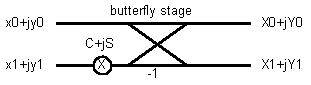The output of a radix-2 complex butterfly stage is: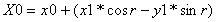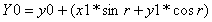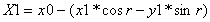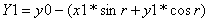Since the complex multiply product is identical for the upper and lower pairs of equations, the four equations can be solved by one complex multiply, one add, and one subtract.

### 2.1. Complex FFT Computation Flow

The general flow of the butterfly computation is:

1. Pre-compute and store values of COS and SIN in packed-word format.
2. Perform a complex multiply accumulate, using the PMADDWD instruction.
3. Pack the real and imaginary products of the complex multiply into 16-bit values.
4. Copy the packed real and imaginary products and reverse their sign.
5. Use the PADDSW instruction to compute the four outputs simultaneously.

A detailed flow of this butterfly computation using MMX instructions is illustrated in Figure 2.

Figure 2. Complex Radix-2 FFT Butterfly Stage Computation Flow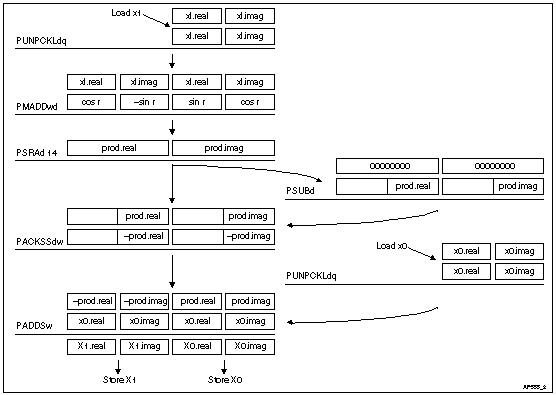In Figure 2, the data at each butterfly stage is stored in packed 16-bit complex pairs with a fractional decimal format of S1.14. The two PUNPCKLDQ instructions expand the input data values, X0 and X1, from packed doublewords to quadwords for the purpose of the complex arithmetic (i.e., to prepare real and imaginary values for result X0 and result X1). After X1 is expanded, the PMADDWD instruction multiplies the result by a packed twiddle factor corresponding to that butterfly stage.

The PSRAD instruction arithmetically shifts the complex product pair down to the lower word, retaining the sign, and scales it simultaneously to restore the fractional decimal format to S1.14. Since a right shift by 16 is required to move data down by one word, and a left shift by 2 is required to move the decimal point from S3.28 to S1.14, the net shift to the right is 14. Note that, since the numeric format may vary for different applications, the actual shift counts used should be adjusted accordingly.

After the product is scaled, the sign of one copy of the packed value is reversed using the PSUBD instruction. The two registers are then packed together as four 16-bit quantities using the PACKSSDW instruction. At this point, the sign of the upper doubleword of the packed product is reversed with respect to the lower doubleword. The PACKSSDW instruction also saturates the signed results as part of the precision conversion back to 16-bits.

The PADDSW instruction computes the final result of the butterfly stage by adding the packed quadword value from the PACKSSDW operation to the packed complex version of X0. The high doubleword contains the complex X1 pair, while the lower doubleword contains the complex X0 pair. Depending on the organization of the FFT data, this may be stored to a single contiguous location using MOVQ or to separate locations using the instruction sequence: MOVD, PSRLQ, MOVD.

## 3.0. OPTIMIZATION OPPORTUNITIES FOR THE PENTIUM® PROCESSOR

When performing a complex FFT algorithm, a multiple and even number of butterfly computations are required at each stage. Instruction scheduling can be optimized by interleaving the instruction sequence so that two butterfly stages are computed in parallel. This technique practically eliminates instruction pairing difficulties and data dependencies with respect to the Pentium® processor. The code example shown in Section 4.0 has a throughput of about 7.5 clocks per butterfly stage, excluding address index calculation and loop control overhead.

Additional optimization techniques, which are general to all Pentium processor-based code, include improving the hit rate of the input data in the CPU's primary cache, adjusting the address alignment of the data and associated twiddle factors, and enhancing the ability of the CPU's primary cache to absorb data during the write back of the results.

To improve the hit rate of the input data, the programmer should determine the optimal way to schedule the FFT routine relative to the way the original input data was derived.

To align data and twiddle factor addresses, allocate 4-byte or 8-byte aligned storage at each stage, depending on the corresponding width of the read or write operation. In Section 4.0, the complex data at each stage should be 4-byte aligned and the packed complex twiddle factors should be 8-byte aligned.

The third optimization goal is to improve the write hit rate to the primary cache when storing the output data from the butterfly stages. For the code in Section 4.0, the stores at the end are grouped together as four separate writes. If these writes miss the cache, they may back up in the processor's write buffer and stall subsequent instructions. The writes may also stall due to the strong ordering requirements of the Pentium processor, which block any write to an E or M state line (i.e. MESI) in the primary cache when a write is active in the processor's write buffers or on the external bus. To avoid this, the programmer should try to reuse the same physical data space as previous invocations of the FFT algorithm. This improves the likelihood that entries have already been allocated in the cache to the same addresses as the writes.

## 4.0. COMPLEX FFT CODE LISTING

```
; /***********************************************************************
; *
; *  Radix-2 Complex FFT (Fast Fourier Transform) routine
; *  in fractional decimal (1.15) using integer data types
; *  (SIMD version)
; *
; *  This routine implements a decimation-in-time, radix-2,
; *  N point FFT.
; *
; *  The input data set is assumed to be in linear order.  This
; *  input data is copied to a separate output data space in
; *  bit reversed order.  The final result then comes
; *  out in linear order in the output data set.
; *
; *    Xr0 = xr0 + xr1*C + xi1*S
; *    Xi0 = xi0 - xr1*S + xi1*C
; *    Xr1 = xr0 - xr1*C - xi1*S
; *    Xi1 = xi0 + xr1*S - xi1*C
; *
; *  where:
; *    xr0 + jxi0 ------------------ + ---------> Xr0 + jXi0
; *                            \    ^
; *                             \  /
; *                              \/
; *                              /\
; *                   C + jS    /  \
; *                            /    v
; *    xr1 + jxi1 ----- X ---------- + ---------> Xr1 + jXi1
; *                              -1
; *
; *  Multiple stage butterfly structure assumes a reverse ordered
; *
; *  For the purpose of overflow protection, input data
; *  should be stored with only 14 bit of precision (b13-b0, b15 = sign).
; *  This allows for the inverse output to be computed without saturating
; *  any values.  For better understood input data, it may be possible to
; *  use all 15 bits of precision.
; *
; *  In addition, this algorithm will temporarily shift input data
; *  by >>1 prior to the arithmetic to avoid local
; *  overflows.  The routine then performs a <<1 at the completion to
; *  restore the output data to the same scale.  As a result, the
; *  significance of b0 is lost.
; *
; *  input values:
; *    din_ptr = pointer to packed input data
; *              (format: Dr  Di  Dr  Di) (i.e. MSDW = LSDW)
; *  sincos_ptr = pointer to precomputed packed sin/cos coefficient table
; *                 (format:  cos  sin -sin  cos)
; *  nsincos_ptr = pointer to precomputed packed sin/cos coefficient table
; *                 (format: -cos -sin  sin -cos)
; *    bitrev_ptr = pointer to pre-calculated bit reversed table
; *    idout_ptr = pointer to packed output inverse data
; *                (format: Dr  Di  Dr  Di)
; *    L = log2(# of points) (i.e. L=6 for 64 points)
; *
; *  output values:
; *    changes contents pointed to by these variables:
; *    idout_ptr = pointer to packed output inverse data
; *
; *  globals used:
; *    PW64 packed_sincos[2^L]
; *    PW64 packed_nsincos[2^L]
; *
; ************************************************************************/
.586

include iammx.inc

ASSUME ds:FLAT, cs:FLAT, ss:FLAT

;************************************************************************
;    Code Segment Declarations
;************************************************************************
;              sincos_ptr, nsincos_ptr,
;              bitrev_ptr,
;              idout_ptr,
;              L);
;

;radix2_cfft Proc Near C din_data:PTR DWORD, sincos_ptr:PTR DWORD,
;	nsincos_ptr:PTR DWORD, bitrev_ptr:PTR DWORD, idout_ptr:PTR DWORD,
;	L:DWORD

_TEXT SEGMENT DWORD PUBLIC USE32 'CODE'

mov		eax, 4[esp]
mov		edx, 8[esp]

push	ebp
push	edi
push	esi
push	ebx
mov		edi, eax
sub		esp, 72			; 0x48
mov		eax, 1
mov		ecx, 112[esp]

mov		8[esp], edx
shl		eax, cl			; N = 2^L
sub		ebx, ebx
mov		20[esp], eax

;first stage of FFT routine
;(handled separately to facilitate bit reverse addressing)
; stage= 1
mov		eax, 20[esp]
cmp		ebx, 20[esp]

;     /* group processing loop
;        i.e. for N=32:
;             stage  n1   n2   coeff_offset    k           i
;               1     2    1       16       1          0,2,4,..30
;     */
;    /* unroll 1st loop to eliminate multiplies by sin,cos
;       since phi=0 */
;     i= 0;
;
;     while (i < N)
mov		[esp], eax
jge	L2
; next line added to manually allocate edx to loop count check value
mov		edx, 20[esp]
mov		esi, 104[esp]

;	   n3= i+1;  /* pointer to 2nd half */
lea	ebp, 1[ebx]
;	   o1= *(bitrev_ptr+i);	/* get bit reversed addr of top half */
mov		ecx, [esi+ebx+4]

L3:
;     {
;

;	   o2= *(bitrev_ptr+n3);	/* get bit reversed addr of low half */
mov		eax, [esi+ebp+4]

;	   movq_msrcptr_r0(din_ptr+o1);	/* read upper half of input data */
movq	mm0, [edi+ecx+8]

;	   movq_msrcptr_r1(din_ptr+o2);	/* read low half of input data */
movq	mm1, [edi+eax+8]

psraw	mm0, 1

movq	mm2, mm0

;	/* scale data by >>1 to prevent overflow during primary loop of FFT */
psraw	mm1, 1

mov		ecx, 108[esp]

paddw	mm0, mm1	;     /* X0.r = X0.r + X1.r */

mov		24[esp], eax
psubw	mm2, mm1	;     /* X1.r = X0.r - X1.r */
;							/* X0.i = X0.i + X1.i */
;	 movq_r0_mdstptr(idout_ptr+i);	/* save X0 to output array */
movq [ecx+ebx+8], mm0

;							/* X1.i = X0.i - X1.i */
;	 movq_r2_mdstptr(idout_ptr+n3);	/* save X1 to output array */
add		ebx, 2		;  i+= 2;

movq [ecx+ebp+8], mm2

;	 n3= i+1;  /* pointer to 2nd half */
lea	ebp, 1[ebx]
;	 o1= *(bitrev_ptr+i);		/* get bit reversed addr of top half */
mov		ecx, [esi+ebx+4]

cmp		ebx, edx
jl	L3
L2:
;     }  /* end of inner loop */
;
;
;   /* primary loop for stages 2 to L-1 */
;   /* last stage (stage = L) is also handled separately */
;   for (stage= 2; stage < L; stage++)
mov		eax, 2
mov		4[esp], eax
cmp		eax, 112[esp]
jge	L4
mov		esi, 108[esp]

L5:
;   {
;     n1= 1 << stage;         /* 2^stage */
mov		ecx, 4[esp]
mov		eax, 1
sub		edi, edi
shl		eax, cl
mov		28[esp], eax
;     n2= n1 >> 1;            /* n1/2 */
mov		eax, 28[esp]
sar		eax, 1
mov		ebx, [esp]
;     coeff_offset>>= 1;      /* successively /2 */
mov		40[esp], eax
sar		ebx, 1
mov		DWORD PTR 48[esp], 0
;     phi= 0;
mov		[esp], ebx
;
;     /* group processing loop
;        i.e. for N=32:
;             stage  n1   n2   coeff_offset    k           i
;               1     2    1       16       1          0,2,4,..30
;               2     4    2        8       1,2        0,4,8,..28
;               3     8    4        4       1,2,3,4    0,8,16,24
;               4    16    8        2       1,2,..,8   0,16
;               5    32   16        1       1,2,..,16  0
;    */
;    /* unroll 1st loop to eliminate multiplies by sin,cos
;       since phi=0 */
;    k= 1;
;    i= 0;
;
;     while (i < N)
cmp		edi, 20[esp]
jge	L6
; next line added to allocate edx to loop count constant
mov		edx, 20[esp]
; next line added to allocate eax to loop count increment
mov		eax, 28[esp]

;       n3= i+n2;  /* pointer to 2nd half */
mov		ebx, 40[esp]
L7:
;     {
;
;       movq_msrcptr_r0(idout_ptr+i); /* read upper half of prior data */
;       movq_msrcptr_r1(idout_ptr+n3);/* read low half of prior data */

movq mm0, [esi+edi+8]

movq mm1, [esi+ebx+8]

movq mm2, mm0                   ; /* save upper half */

paddw.mm0, mm1                  ; /* X0.r = X0.r + X1.r */

psubw mm2, mm1                  ; /* X1.r = X0.r - X1.r */

;                                         /* X0.i = X0.i + X1.i */
;       movq_r0_mdstptr(idout_ptr+i);     /* save X0 */
movq [esi+edi+8], mm0

;                                         /* X1.i = X0.i - X1.i */
;       movq_r2_mdstptr(idout_ptr+n3)   ; /* save X1 */
movq [esi+ebx+8], mm2

;
;       i+= n1;
cmp	    edi, edx
jl	    L7
L6:
;     }  /* end of inner loop */
;
;     phi+= coeff_offset;
mov	    eax, [esp]
;
mov	    ebx, 2
;     /* 2nd and subsequent passes of inner loop */
;     for (k=2; k<= n2; ++k)
mov	    64[esp], ebx
cmp	    ebx, 40[esp]
jg	    L8

L9:
;     {
;       i= k-1;
mov		edi, 64[esp]
dec		edi
;
;       while (i < N)
cmp		edi, 20[esp]
jge	L10
mov		eax, 48[esp]
mov		ecx, 8[esp]
lea		eax, [ecx+eax+8]
mov		56[esp], eax
mov		eax, 48[esp]
mov		ecx, 100[esp]
lea		eax, [ecx+eax+8]
mov		60[esp], eax
; next line added to allocate edx to 60(%esp)
mov		edx, eax
; next line added to allocate ecx to loop count constant
mov		ecx, 20[esp]
; next line added to allocate ebp to loop count increment
mov		ebp, 28[esp]

mov		eax, 40[esp]
mov	     ebx, 56[esp]
movq	mm6, [ebx]
movq mm7, [edx]

movq	mm1, mm6

L11:
;       {
;         n3= i+n2;  /* pointer to 2nd half */
;
;         movq_msrcptr_r1(sincos_ptr+phi);  /* load sin/cos values */

;         /* X.r' =   x.r * cos + x.i * sin
;            X.i' = - x.r * sin + x.i * cos  */
;pmaddwd	mm1, mm0	;        /* multiply & combine */

;	  movq_msrcptr_r2(nsincos_ptr+phi);	/* load -sin/cos values */
movq	mm2, mm7

;	  movq_msrcptr_r3(idout_ptr+i);	/* load prior top half of data */
movq	mm3, [esi+edi+8]

;            X.i' =   x.r * sin - x.i * cos  */
;pmaddwd	mm2, mm0	;			/* multiply & combine */

;         /* X.r' = - x.r * cos - x.i * sin
;
psrad	mm1, 15		;	/* prepare to get MSW for result #1 */

;                                   /* & adjust for 2.30 -> 1.15 */
packssdw	mm1, mm1	;		/* convert to from packed double */

;                                   /* to duplicate packed word */
paddsw	mm1, mm3	;		/* add result #1 to prior top half */

psrad	mm2, 15		;	/* prepare to get MSW for result #2 */

;
packssdw mm2, mm2	;	/* convert to from packed double */

;                                           /* & adjust for 2.30 -> 1.15 */
;   movq_r1_mdstptr(idout_ptr+i);	/* store data result #1 to top half */
movq [esi+edi+8], mm1

paddsw	mm2, mm3	;		/* add result #2 to prior top half */

;                                           /* to duplicate packed word */
;   movq_r2_mdstptr(idout_ptr+n3);	/* store data result #2 to low half */
movq	[esi+eax+8], mm2

movq	mm1, mm6

cmp		edi, ecx

;         i+= n1;
jl	L11
L10:
;       }  /* end of inner loop */
;
;       phi+= coeff_offset;
mov		eax, [esp]
inc		DWORD PTR 64[esp]
mov		eax, 40[esp]
cmp		64[esp], eax
jle	L9
L8:
mov		eax, 112[esp]
inc		DWORD PTR 4[esp]
cmp		4[esp], eax
jl	L5
L4:
;
;     }  /* end of group loop */
;
;   }  /* end of outer stage loop */
;
;
;   /* last stage is handled separately so the final <<1 with saturation
;      can be done in the loop
;   */
;     stage= L;
;     n1= 1 << stage;         /* 2^stage */
mov		ecx, 112[esp]
mov		eax, 1
shl		eax, cl
mov		32[esp], eax
;     n2= n1 >> 1;            /* n1/2 */
mov		eax, 32[esp]
sar		eax, 1
mov		ebx, [esp]
;     coeff_offset>>= 1;      /* successively /2 */
mov		36[esp], eax
sar		ebx, 1
mov		DWORD PTR 16[esp], 0
;     phi= 0;
mov		eax, 1
;
;     /* inner loop */
;     for (k=1; k<= n2; ++k)
mov		[esp], ebx
mov		12[esp], eax
cmp		eax, 36[esp]
jg	L12
mov		edi, 108[esp]

L13:
;     {
;       i= k-1;
mov		esi, 12[esp]
dec		esi
;

;       while (i < N)
cmp		esi, 20[esp]
jge	L14
mov		ecx, 16[esp]
mov		eax, 8[esp]
lea		eax, [eax+ecx+8]
mov		52[esp], eax
mov		eax, ecx
mov		ecx, 100[esp]
lea		eax, [ecx+eax+8]
mov		44[esp], eax
; next line added to allocate ecx for use as loop count constant
mov		ecx, 20[esp]
; next line added to allocate edx for use as loop count increment
mov		edx, 32[esp]

mov		eax, 36[esp]
mov		ebp, 44[esp]
mov		ebx, 52[esp]
L15:
;       {
;         n3= i+n2;  /* pointer to 2nd half */

;
;         movq_msrcptr_r1(sincos_ptr+phi);  /* load sin/cos values */
movq	mm1, [ebx]

;         movq_msrcptr_r0(idout_ptr+n3);    /* load lower half of data */
movq	mm0, [edi+eax+8]

;         /* X.r' =   x.r * cos + x.i * sin
;            X.i' = - x.r * sin + x.i * cos  */
pmaddwd		mm1, mm0	;	  /* multiply & combine */

;         movq_msrcptr_r2(nsincos_ptr+phi); /* load -sin/cos values */
movq	mm2, [ebp]

;         /* X.r' = - x.r * cos - x.i * sin
;            X.i' =   x.r * sin - x.i * cos  */
pmaddwd		mm2, mm0	;        /* multiply & combine */

;         movq_msrcptr_r3(idout_ptr+i);     /* load prior top half of data */
movq	mm3, [edi+esi+8]

;
psrad		mm1, 15		;	  /* prepare to get MSW for result #1 */

;                                           /* & adjust for 2.30 -> 1.15 */
packssdw	mm1, mm1	;       /* convert to from packed double */

;
psrad		mm2, 15		;	  /* prepare to get MSW for result #2 */

;                                           /* to duplicate packed word */
paddsw		mm1, mm3	;      /* add result #1 to prior top half */

;
psllw	mm1, 1		;           /* undo manual scaling performed */

;                                       /* prior to FFT routine */
;     movq_r1_mdstptr(idout_ptr+i);     /* store data result #1 to top half */
movq	[edi+esi+8], mm1

;                                     /* & adjust for 2.30 -> 1.15 */
packssdw	mm2, mm2	;       /* convert to from packed double */
;                                       /* to duplicate packed word */
paddsw		mm2, mm3	;       /* add result #2 to prior top half */

;
psllw	mm2, 1		;         /* undo manual scaling performed */

cmp		esi, ecx
;                                       /* prior to FFT routine */
;       movq_r2_mdstptr(idout_ptr+n3);  /* store data result #2 to low half */
movq	[edi+eax+8], mm2

mov		eax, 36[esp]
;
;
;         i+= n1;
jl	L15
L14:
;       }  /* end of inner loop */
;
;       phi+= coeff_offset;
mov		eax, [esp]
mov		ebx, 12[esp]
inc		ebx
mov		12[esp], ebx
cmp		ebx, 36[esp]
jle	L13
L12:
;
;     }  /* end of group loop */
;
;
; } /* end of radix2_cfft */